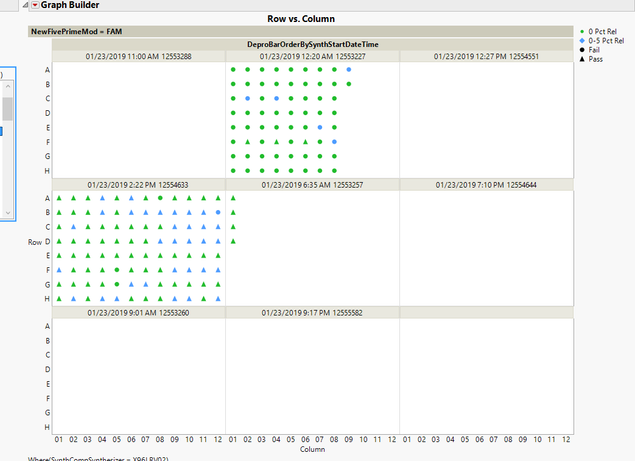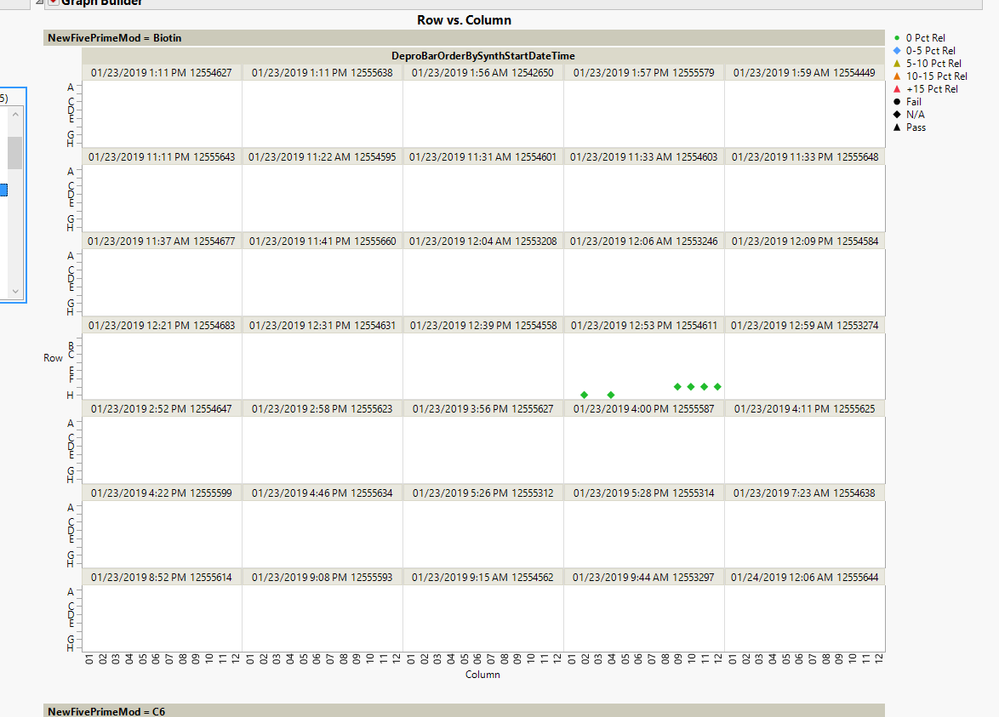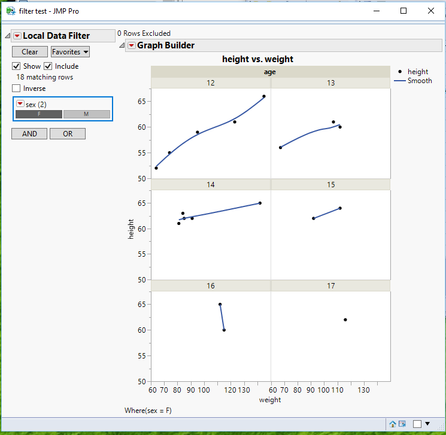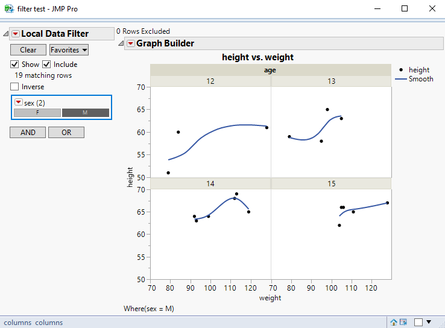Our World Statistics Day conversations have been a great reminder of how much statistics can inform our lives. Do you have an example of how statistics has made a difference in your life? Share your story with the Community!
Choose Language Hide Translation Bar
Highlighted

## Lock Size of Graph Builder with Page and Wrap Variables

Is there a way that I can force the graphs in graph builder to stay the same size? I have an analysis that opens an interactive graph that includes both wrap and page variables. There is a local data filter that allows the user to go look at the data by instrument. However, not every instrument contains data in all of the categories of the page variable. I can't figure out how to keep the graphs from automatically resizing when the user selects a different option from the local data filter. For example, this instrument has data in only one of the categories of the page variable:This data is exactly how I would like to see it, with each individual graph (from the wrap variable) sized to isometric. When I select a different instrument from the local data filter, one that has data in multiple categories of the page variable, the graphs can become difficult see:Is there anything I can add to my script to make it so that each graph stays relatively the same size no matter how many categories of the wrap or page variables appear?

2 REPLIES 2
Highlighted

## Re: Lock Size of Graph Builder with Page and Wrap Variables

Here is an example based on the Big Class data table that does what I think you want.``````Names Default To Here( 1 );
dt = Open( "\$SAMPLE_DATA/Big Class.jmp" );

// make the number of graphs different for males
dt << select where( :sex == "M" & :age >=16 );
dt << delete rows;

New Window( "filter test",
Data Filter Context Box(
H List Box(
dt << Data Filter(
Local,
columns( :sex )
),
Mode( Select( 0 ), Show( 1 ), Include( 1 ) )
),
V List Box(
t = Text Box( "0 Rows Excluded" ),
gb =Graph Builder(
Size( 528, 490 ),
Show Control Panel( 0 ),
Variables( X( :weight ), Y( :height ), Wrap( :age ) ),
Elements( Points( X, Y, Legend( 27 ) ), Smoother( X, Y, Legend( 28 ) ) )
)
)
)
)
);
updatetext = Function( {},
rs = t << Get Row States();
n = 0;
For( ii = 1, ii <= N Rows( rs ), ii++,
If( Excluded( As Row State( rs[ii] ) ),
n
++)
);
t << Set Text( Char( n ) || " Rows Excluded" );
);
rsupdate = Function( {a},
(gb << xpath( "//FrameBox") ) << frame size( 200,150);
);
rsh = t << Make Row State Handler( dt, rsupdate );
updatetext();``````
Jim
Highlighted

## Re: Lock Size of Graph Builder with Page and Wrap Variables

Oops...I didn't see the sample output.  But all you have to do to make that happen, is to make your data table have at least one row for each combination of your wrap column.  Here is an example of that:

``````Names Default To Here( 1 );
dt = Open( "\$SAMPLE_DATA/Big Class.jmp" );

// make the number of graphs different for males but has rows with missing data
dt << select where( :sex == "M" & :age >=16 );
dt:height[dt << get selected rows] = .;

New Window( "filter test",
Data Filter Context Box(
H List Box(
dt << Data Filter(
Local,
columns( :sex )
),
Mode( Select( 0 ), Show( 1 ), Include( 1 ) )
),
V List Box(
t = Text Box( "0 Rows Excluded" ),
gb =Graph Builder(
Size( 528, 490 ),
Show Control Panel( 0 ),
Variables( X( :weight ), Y( :height ), Wrap( :age ) ),
Elements( Points( X, Y, Legend( 27 ) ), Smoother( X, Y, Legend( 28 ) ) )
)
)
)
)
);``````
Jim
Article Labels

There are no labels assigned to this post.